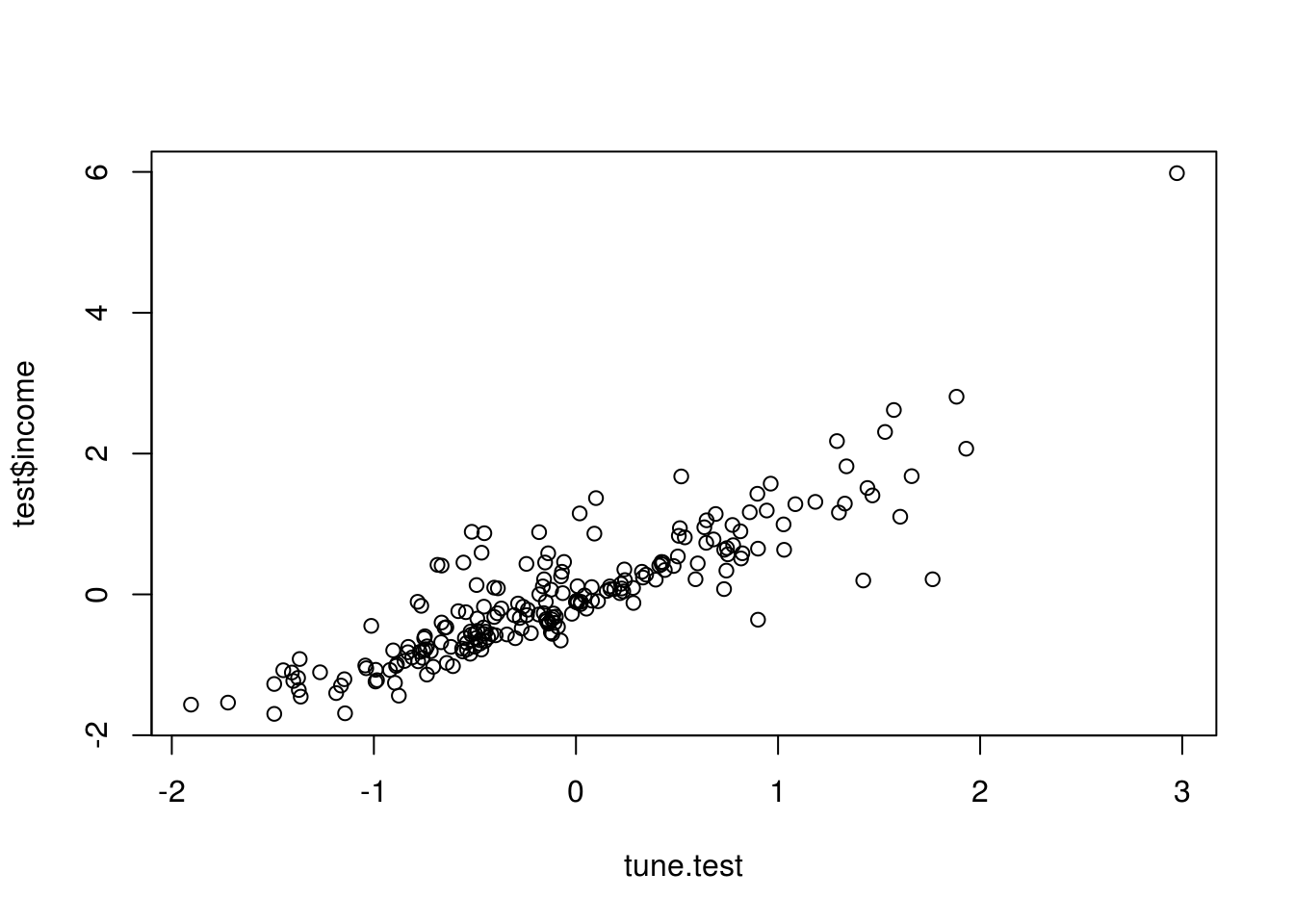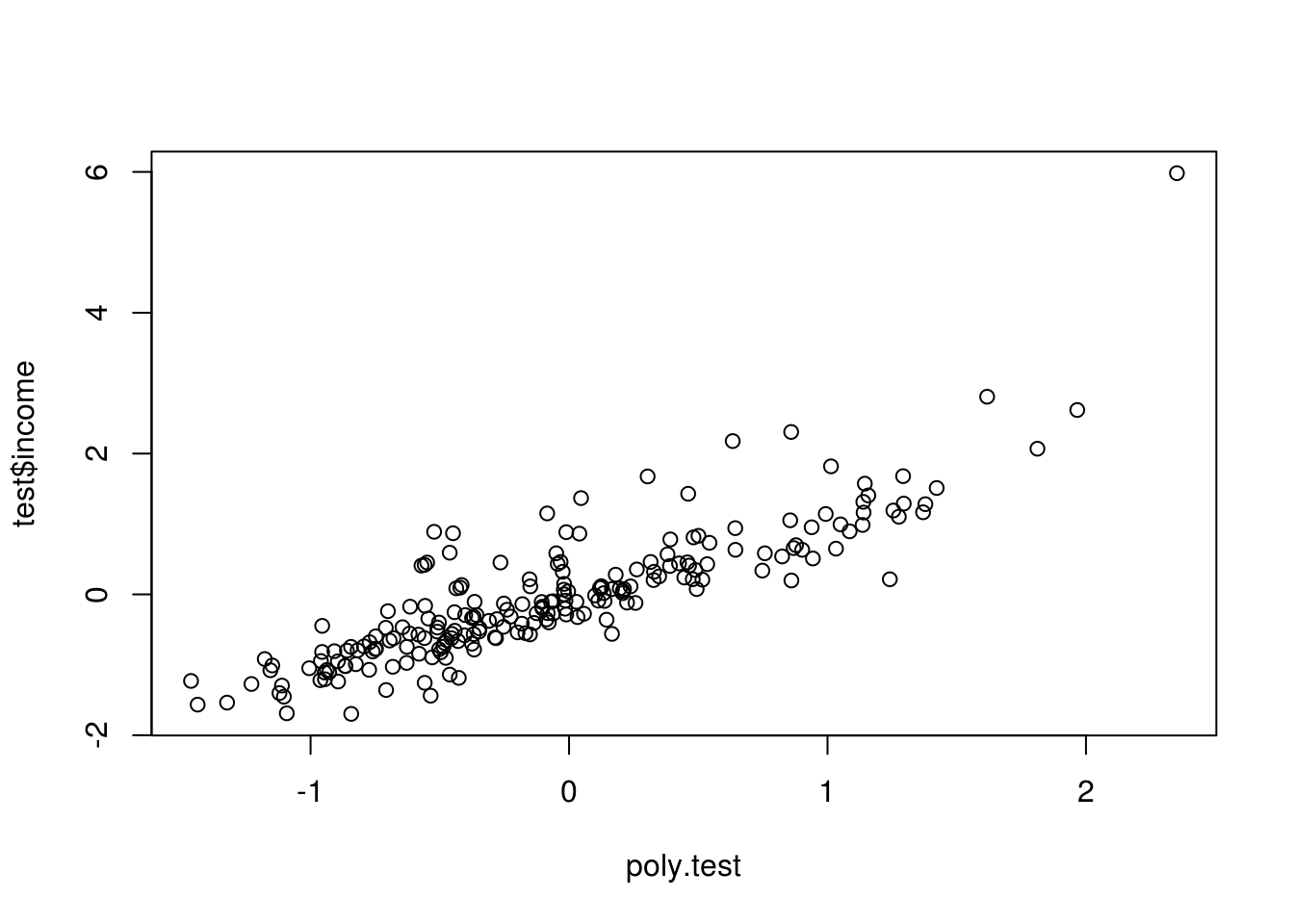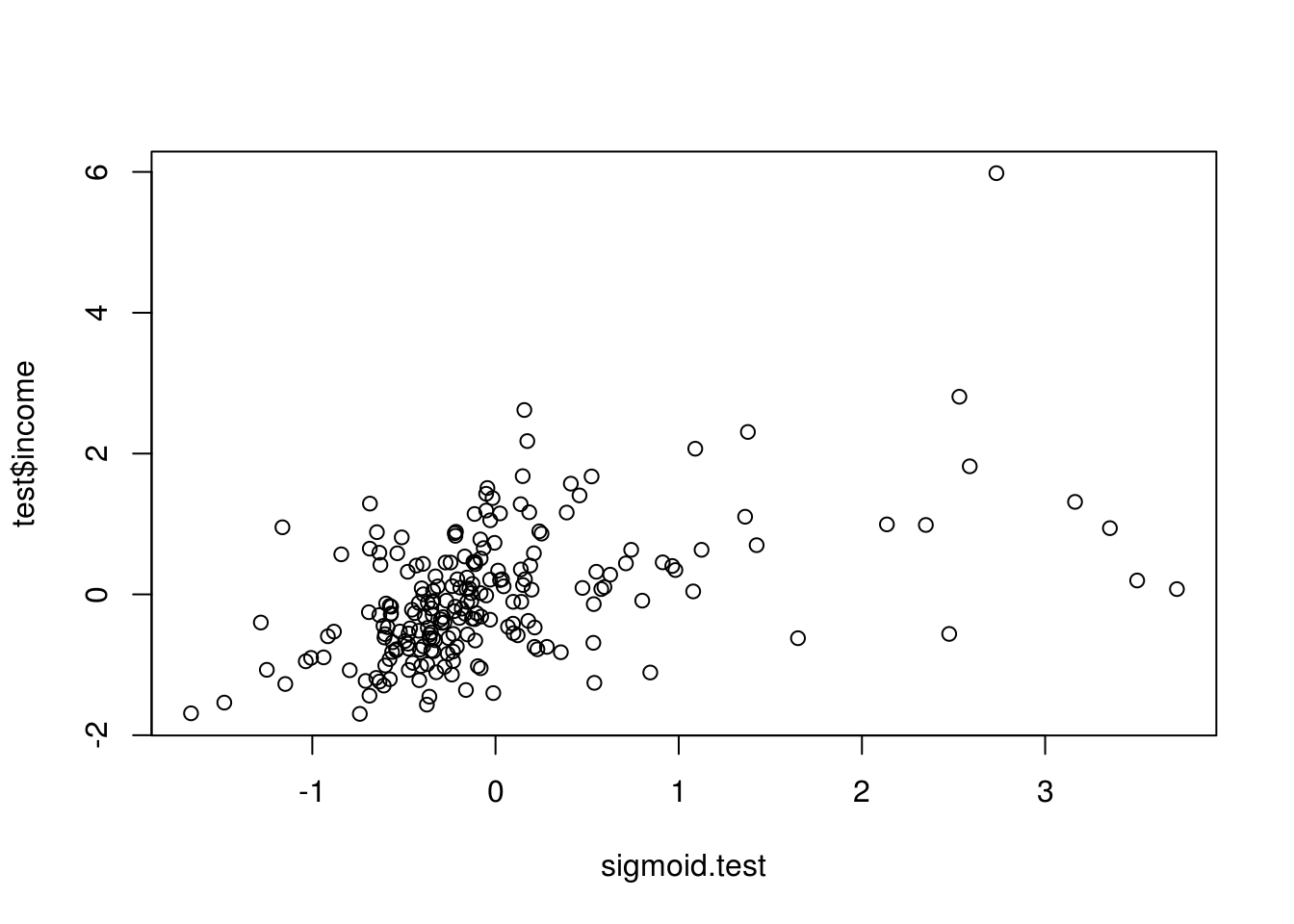# Numeric Prediction with Support Vector Machines in R

In this post, we will look at support vector machines for numeric prediction. SVM is used for both classification and numeric prediction. The advantage of SVM for numeric prediction is that SVM will automatically create higher dimensions of the features and summarizes this in the output. In other words, unlike in regression where you have to decide for yourself how to modify your features, SVM does this automatically using different kernels.

Different kernels transform the features in different ways. And the cost function determines the penalty for an example being on the wrong side of the margin developed by the kernel. Remember that SVM draws lines and separators to divide the examples. Examples on the wrong side are penalized as determined by the researcher.

Just like with regression, generally, the model with the least amount of error may be the best model. As such, the purpose of this post is to use SVM to predict income in the “Mroz” dataset from the “Ecdat” package. We will use several different kernels that will transformation the features different ways and calculate the mean-squared error to determine the most appropriate model. Below is some initial code.

``library(caret);library(e1071);library(corrplot);library(Ecdat)``
``````data(Mroz)
str(Mroz)``````
``````## 'data.frame':    753 obs. of  18 variables:
##  \$ work      : Factor w/ 2 levels "yes","no": 2 2 2 2 2 2 2 2 2 2 ...
##  \$ hoursw    : int  1610 1656 1980 456 1568 2032 1440 1020 1458 1600 ...
##  \$ child6    : int  1 0 1 0 1 0 0 0 0 0 ...
##  \$ child618  : int  0 2 3 3 2 0 2 0 2 2 ...
##  \$ agew      : int  32 30 35 34 31 54 37 54 48 39 ...
##  \$ educw     : int  12 12 12 12 14 12 16 12 12 12 ...
##  \$ hearnw    : num  3.35 1.39 4.55 1.1 4.59 ...
##  \$ wagew     : num  2.65 2.65 4.04 3.25 3.6 4.7 5.95 9.98 0 4.15 ...
##  \$ hoursh    : int  2708 2310 3072 1920 2000 1040 2670 4120 1995 2100 ...
##  \$ ageh      : int  34 30 40 53 32 57 37 53 52 43 ...
##  \$ educh     : int  12 9 12 10 12 11 12 8 4 12 ...
##  \$ wageh     : num  4.03 8.44 3.58 3.54 10 ...
##  \$ income    : int  16310 21800 21040 7300 27300 19495 21152 18900 20405 20425 ...
##  \$ educwm    : int  12 7 12 7 12 14 14 3 7 7 ...
##  \$ educwf    : int  7 7 7 7 14 7 7 3 7 7 ...
##  \$ unemprate : num  5 11 5 5 9.5 7.5 5 5 3 5 ...
##  \$ city      : Factor w/ 2 levels "no","yes": 1 2 1 1 2 2 1 1 1 1 ...
##  \$ experience: int  14 5 15 6 7 33 11 35 24 21 ...``````

We need to place the factor variables next to each other as it helps in having to remove them when we need to scale the data. We must scale the data because SVM is based on distance when making calculations. If there are different scales the larger scale will have more influence on the results. Below is the code

``````mroz.scale<-Mroz[,c(17,1,2,3,4,5,6,7,8,9,10,11,12,13,14,15,16,18)]
mroz.scale<-as.data.frame(scale(mroz.scale[,c(-1,-2)])) #remove factor variables for scaling
mroz.scale\$city<-Mroz\$city # add factor variable back into the dataset
mroz.scale\$work<-Mroz\$work # add factor variable back into the dataset
#mroz.cor<-cor(mroz.scale[,-17:-18])
#corrplot(mroz.cor,method='number', col='black')``````

Below is the code for creating the train and test datasets.

``````set.seed(502)
ind=sample(2,nrow(mroz.scale),replace=T,prob=c(.7,.3))
train<-mroz.scale[ind==1,]
test<-mroz.scale[ind==2,]``````

Linear Kernel

Our first kernel is the linear kernel. Below is the code. We use the “tune.svm” function from the “e1071” package. We set the kernel to “linear” and we pick our own values for the cost function. The numbers for the cost function can be whatever you want. Also, keep in mind that r will produce six different models because we have six different values in the “cost” argument.

The process we are using to develop the models is as follows

1. Set the seed
2. Develop the initial model by setting the formula, dataset, kernel, cost function, and other needed information.
3. Select the best model for the test set
4. Predict with the best model
5. Plot the predicted and actual results
6. Calculate the mean squared error

The first time we will go through this process step-by-step. However, all future models will just have the code followed by an interpretation.

``````linear.tune<-tune.svm(income~.,data=train,kernel="linear",cost = c(.001,.01,.1,1,5,10))
summary(linear.tune)``````
``````##
## Parameter tuning of 'svm':
##
## - sampling method: 10-fold cross validation
##
## - best parameters:
##  cost
##    10
##
## - best performance: 0.3492453
##
## - Detailed performance results:
##    cost     error dispersion
## 1 1e-03 0.6793025  0.2285748
## 2 1e-02 0.3769298  0.1800839
## 3 1e-01 0.3500734  0.1626964
## 4 1e+00 0.3494828  0.1618478
## 5 5e+00 0.3493379  0.1611353
## 6 1e+01 0.3492453  0.1609774``````

The best model had a cost = 10 with a performance of .35. We will select the best model and use this on our test data. Below is the code.

``````best.linear<-linear.tune\$best.model
tune.test<-predict(best.linear,newdata=test)``````

Now we will create a plot so we can see how well our model predicts. In addition, we will calculate the mean squared error to have an actual number of our model’s performance

``plot(tune.test,test\$income)````````tune.test.resid<-tune.test-test\$income
mean(tune.test.resid^2)``````
``##  0.215056``

The model looks good in the plot. However, we cannot tell if the error number is decent until it is compared to other models

Polynomial Kernel

The next kernel we will use is the polynomial one. The kernel requires two parameters the degree of the polynomial (3,4,5, etc) as well as the kernel coefficient. Below is the code

``````set.seed(123)
poly.tune<-tune.svm(income~.,data = train,kernal="polynomial",degree = c(3,4,5),coef0 = c(.1,.5,1,2,3,4))
best.poly<-poly.tune\$best.model
poly.test<-predict(best.poly,newdata=test)
plot(poly.test,test\$income)````````````poly.test.resid<-poly.test-test\$income
mean(poly.test.resid^2)``````
``##  0.2453022``

The polynomial has an insignificant additional amount of error.

Next, we will use the radial kernel. One thing that is new here is the need for a parameter in the code call gamma. Below is the code.

``````set.seed(123)
summary(rbf.tune)``````
``````##
## Parameter tuning of 'svm':
##
## - sampling method: 10-fold cross validation
##
## - best parameters:
##  gamma
##    0.1
##
## - best performance: 0.5225952
##
## - Detailed performance results:
##   gamma     error dispersion
## 1   0.1 0.5225952  0.4183170
## 2   0.5 0.9743062  0.5293211
## 3   1.0 1.0475714  0.5304482
## 4   2.0 1.0582550  0.5286129
## 5   3.0 1.0590367  0.5283465
## 6   4.0 1.0591208  0.5283059``````
``````best.rbf<-rbf.tune\$best.model
rbf.test<-predict(best.rbf,newdata=test)
plot(rbf.test,test\$income)````````````rbf.test.resid<-rbf.test-test\$income
mean(rbf.test.resid^2)``````
``##  0.3138517``

The radial kernel is worst than the linear and polynomial kernel. However, there is not much different in the performance of the models so far.

Sigmoid Kernel

Next, we will try the sigmoid kernel. Sigmoid kernel relies on a “gamma” parameter and a cost function. Below is the code

``````set.seed(123)
sigmoid.tune<-tune.svm(income~., data=train,kernel="sigmoid",gamma = c(.1,.5,1,2,3,4),coef0 = c(.1,.5,1,2,3,4))
summary(sigmoid.tune)``````
``````##
## Parameter tuning of 'svm':
##
## - sampling method: 10-fold cross validation
##
## - best parameters:
##  gamma coef0
##    0.1     3
##
## - best performance: 0.8759507
##
## - Detailed performance results:
##    gamma coef0        error  dispersion
## 1    0.1   0.1   27.0808221   6.2866615
## 2    0.5   0.1  746.9235624 129.0224096
## 3    1.0   0.1 1090.9660708 198.2993895
## 4    2.0   0.1 1317.4497885 214.7997608
## 5    3.0   0.1 1339.8455047 180.3195491
## 6    4.0   0.1 1299.7469190 201.6901577
## 7    0.1   0.5  151.6070833  38.8450961
## 8    0.5   0.5 1221.2396575 335.4320445
## 9    1.0   0.5 1225.7731007 190.7718103
## 10   2.0   0.5 1290.1784238 216.9249899
## 11   3.0   0.5 1338.1069460 223.3126800
## 12   4.0   0.5 1261.8861304 300.0001079
## 13   0.1   1.0  162.6041229  45.3216740
## 14   0.5   1.0 2276.4330973 330.1739559
## 15   1.0   1.0 2036.4791854 335.8051736
## 16   2.0   1.0 1626.4347749 290.6445164
## 17   3.0   1.0 1333.0626614 244.4424896
## 18   4.0   1.0 1343.7617925 194.2220729
## 19   0.1   2.0   19.2061993   9.6767496
## 20   0.5   2.0 2504.9271757 583.8943008
## 21   1.0   2.0 3296.8519140 542.7903751
## 22   2.0   2.0 2376.8169815 398.1458855
## 23   3.0   2.0 1949.9232179 319.6548059
## 24   4.0   2.0 1758.7879267 313.2581011
## 25   0.1   3.0    0.8759507   0.3812578
## 26   0.5   3.0 1405.9712578 389.0822797
## 27   1.0   3.0 3559.4804854 843.1905348
## 28   2.0   3.0 3159.9549029 492.6072149
## 29   3.0   3.0 2428.1144437 412.2854724
## 30   4.0   3.0 1997.4596435 372.1962595
## 31   0.1   4.0    0.9543167   0.5170661
## 32   0.5   4.0  746.4566494 201.4341061
## 33   1.0   4.0 3277.4331302 527.6037421
## 34   2.0   4.0 3643.6413379 604.2778089
## 35   3.0   4.0 2998.5102806 471.7848740
## 36   4.0   4.0 2459.7133632 439.3389369``````
``````best.sigmoid<-sigmoid.tune\$best.model
sigmoid.test<-predict(best.sigmoid,newdata=test)
plot(sigmoid.test,test\$income)````````````sigmoid.test.resid<-sigmoid.test-test\$income
mean(sigmoid.test.resid^2)``````
``##  0.8004045``

The sigmoid performed much worst then the other models based on the metric of error. You can further see the problems with this model in the plot above.

Conclusion

The final results are as follows

• Linear kernel .21
• Polynomial kernel .24